# Vector bundle, analytic

(diff) ← Older revision | Latest revision (diff) | Newer revision → (diff)

A locally trivial analytic bundle over an analytic space whose fibres have the structure of an-dimensional vector space over a ground field(if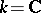is the field of complex numbers, the analytic bundle is said to be holomorphic). The numberis said to be the dimension, or rank, of the bundle. Similarly as for a topological bundle (cf. Vector bundle), definitions are given of the category of analytic vector bundles, and of the concepts of a subbundle, a quotient bundle, the direct sum, the tensor product, the exterior product of analytic vector bundles, etc.

The analytic sections of an analytic vector bundle with baseform a moduleover the algebraof analytic functions on the base. Ifandis compact,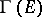is a finite-dimensional vector space over(see Finiteness theorems). If, on the other hand,is a finite-dimensional complex Stein space, thenis a projective module of finite type over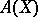, and the correspondence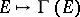defines an equivalence between the category of analytic vector bundles overand the category of projective-modules of finite type .

Examples of analytic vector bundles include the tangent bundle of an analytic manifold(its analytic sections are analytic vector fields on), and the normal bundle of a submanifold.

The classification of analytic vector bundles of rankon a given analytic spaceis equivalent with the classification of principal analytic fibrations (cf. Principal analytic fibration) with baseand structure groupand, for, has been completed only in certain special cases. For projective complex algebraic varietiesit is identical with the classification of algebraic vector bundles (cf. Comparison theorem (algebraic geometry)).

Analytic vector bundles of rank 1 on a complex space(in other words, bundles of complex lines, or line bundles) play an important part in complex analytic geometry. Each divisor on the spacenecessarily defines an analytic bundle of rank 1, two divisors defining isomorphic bundles if and only if they are linearly equivalent. All analytic line bundles on a projective algebraic variety are defined by a divisor. The imbeddability of a complex spaceinto a projective space is closely connected with the existence of ample line bundles on(cf. Ample vector bundle). If one is given a discrete groupof automorphisms of a complex space, each quotient ofwill determine a line bundle over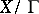, with the respective automorphic forms as its analytic sections. Analytic vector bundles of rank 1 constitute the group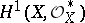, where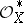is the sheaf of invertible elements of the structure sheaf. The correspondence between each bundle and its first Chern class yields the homomorphismwhose kernel is the set of topologically trivial line bundles. Ifis a complex manifold,may be described as the set of cohomology classes which are representable by closed differential forms of type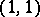. If, in addition,is compact and Kählerian,is isomorphic to the Picard variety of the manifoldand is thus a complex torus .

To each analytic vector bundleof rankon an analytic spacecorresponds a sheaf of germs of analytic sections of, which is a locally free analytic sheaf of rankon. This correspondence defines an equivalence between the categories of analytic vector bundles and locally free analytic sheaves on. Attempts to generalize this result to arbitrary coherent analytic sheaves resulted in the following generalization of the concept of an analytic vector bundle : A surjective morphismis said to be an analytic family of vector spaces over(or a linear space over) if its fibres have the structure of finite-dimensional vector spaces over, and if the operations of addition, multiplication by a scalar and the zero section satisfy the natural conditions of analyticity. If(orandis coherent), the analytic family of vector spaces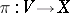defines a coherent analytic sheafon: For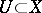the group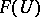is the space of analytic functions on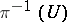which are linear on the fibres. In the same way is defined the duality between the categories of analytic families of vector spaces and coherent analytic sheaves on.

How to Cite This Entry:
Vector bundle, analytic. Encyclopedia of Mathematics. URL: http://encyclopediaofmath.org/index.php?title=Vector_bundle,_analytic&oldid=13046
This article was adapted from an original article by A.L. Onishchik (originator), which appeared in Encyclopedia of Mathematics - ISBN 1402006098. See original article